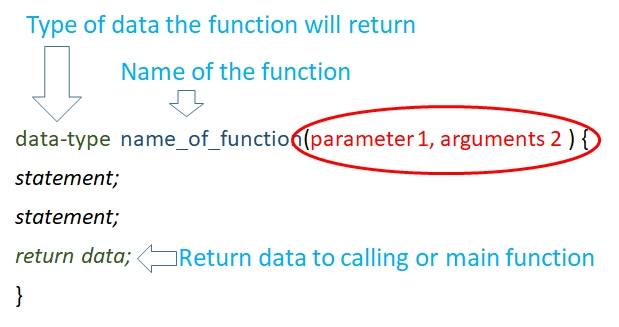# Functions in C

C has several built-in library functions , one of them we frequently use are scanf()& printf(). These function are kept inside <stdio.h> and called at the starting of the page. In addition to these library function which C provides us, we will learn about User defined Functions ( UDF ) here .

Using functions we can avoid repeated codes blocks doing the same job. This also modularize the script helping in easy maintenance . Functions does some specific works and return to calling script once the task is over.While declaring function we have to declare data type based on the data the function is going to return.
Function name: We can’t use coma or space or special characters for using function name
We can pass variables to the function and get return value to main or calling function.

## Average of two numbers

``````#include <stdio.h>
float find_average(float num1,float num2)
{
float avg ;
avg=(num1+num2)/2;
return avg;
}
int main()
{
int i=10,j=11;
float final_avg;
final_avg=find_average(i,j);
printf("Average =%f ",final_avg);
return 0;
}``````
Output is here
``Average =10.500000``

## Lower case letter to Upper case letter

We are not using strupr() library function
``````#include <stdio.h>
char lower_to_upper_case(char str1)
{
char str2;
if(str1 >='a' && str1<='z'){
str2='A'+str1-'a';
}else{str2=str1;}
return str2;
}
int main()
{
char lower_str,upper_str;
printf("Enter a case in lower char ");
scanf("%c",&lower_str);
upper_str=lower_to_upper_case(lower_str);
printf("The upper case char is  = %c ",upper_str);
return 0;
}``````

## Passing a pointer to a function

Basic salary increased by 15% , User defined function ( UDF ) should return the new salary after receiving the basic salary.
``````#include <stdio.h>
int salary(int *basic){
int final_sal=0;
final_sal=*basic*1.5;
return final_sal;
}
int main(void){
int *pot, sal,final_sal;

sal=1000;
pot=&sal; // address is stored in pointer
final_sal=salary(pot); // Passed the pointer as parameter  to the function
printf("Final Salary =%d ",final_sal);
}``````
Output is here
``Final Salary =1500``

## Passing array as input parameter to a function

We will find out sum of the elements of an input array inside a function and return the sum to main function.
``````#include <stdio.h>
int array_sum(int ar[]){
int k,sum=0;
for(k=0;k<5;k++){
sum = sum+ ar[k];
}
//sum=ar;
return sum;
}
int main(void){
int j={1,2,3,4,5};
int sum;
sum=array_sum(j);
printf("Sum = %d \n",sum);
return 0;
}``````
Output is here
``Sum =15``

## Passing pointer of an array as parameter to a function

We will pass the pointer storing the address of first element of the array. Inside the function we will increase the pointer to read all other elements of the array. As an additional parameter we can pass size of the array to the function.
``````#include <stdio.h>
int array_sum(int *pot){
int k,sum=0;
for(k=0;k<5;k++){
sum = sum+ *pot;
pot++;
}
//sum=ar;
return sum;
}
int main(void){
int j={1,2,3,4,5};
int sum;
int *pot;
pot=&j;
sum=array_sum(pot);
printf("Sum = %d \n",sum);
return 0;
}``````
Your Rating

Post your comments , suggestion , error , requirements etc here

We use cookies to improve your browsing experience. . Learn more
 HTML MySQL PHP JavaScript ASP Photoshop Articles FORUM . Contact us
©2000-2019 plus2net.com All rights reserved worldwide Privacy Policy Disclaimer